Graph all the things

analyzing all the things you forgot to wonder about

# Rivers

2022-04-14

Thought experiment: if a riverbed of constant width rises as you move downstream, how can the river's height and speed change? (choose any subset, answer at bottom of page)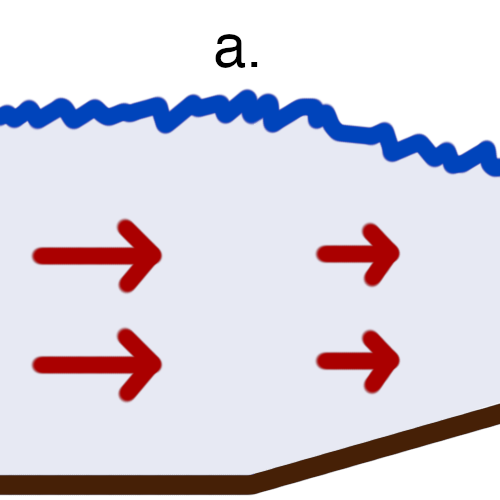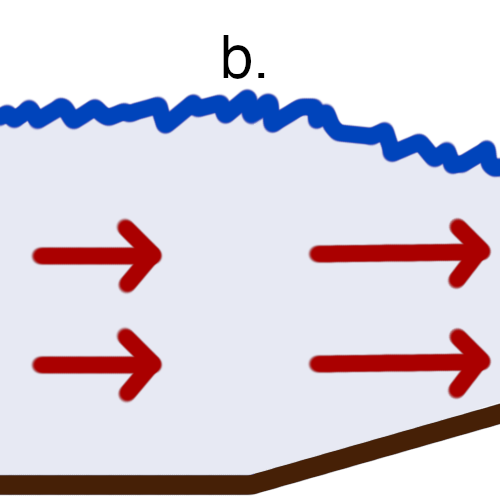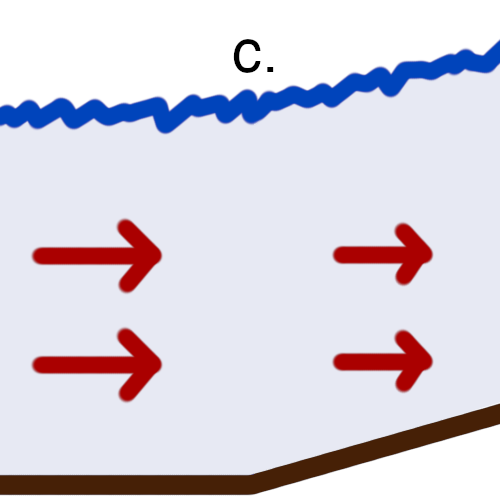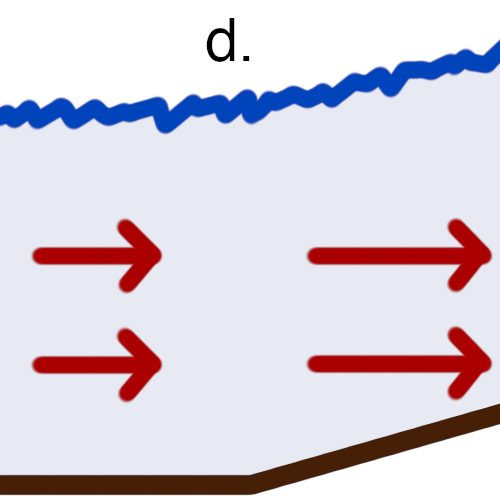1. top of river drops, speed decreases
2. top of river drops, speed increases
3. top of river rises, speed decreases
4. top of river rises, speed increases

I made a model of river depth and speed, given riverbed altitude and flow, that explains the thought experiment and more, including river reversals!

You can play around with it in the visualization below - drag the riverbed nodes to change the riverbed altitude.

Initial Speed: 1 m/s    5 m/s

This visualization uses a Runge-Kutta 4th order method with built-in criticality detection (more on criticality later). It assumes a 15m wide river with 70m3/s discharge (think Rio Grande). If your river doesn't make it to the end, then it isn't a possible river; water would build up at the start. Try reducing the initial water speed.

If you're careful, you can create both pools and fast-moving water in the same river by crossing the critical velocity line right as the riverbed slope angles downward.

### Backstory

I've always been interested in flowing water, and almost a decade ago I figured I could calculate a river's depth from its riverbed. Somehow I repeatedly fumbled the derivation and eventually gave up, only to revisit it recently.

Of course, there are many well-known fluid dynamics equations and simulation techniques. But many of these are taught as special cases of more esoteric equations like Navier-Stokes. Instead of working esoteric-down, I wanted to derive something interesting from first-principles-up.

After finishing my own derivations, I did some research and was pleasantly surprised to find my equations were equivalent to the Saint-Venant equations (save a factor of 2 I needed to fix).

### River Physics

You only need high school mechanics understand what's going on in a river. Three forces act on the water:

• gravity, which converts the water's potential energy into kinetic energy;
• contact force (or water pressure), which causes parts of the water to push or pull on nearby water; and
• friction, which converts the water's kinetic energy into other things like heat.

We'll ignore viscosity, so the only important difference between a liquid and solid for this problem is that when a liquid is under pressure, it applies that pressure in all directions.

To keep things simple, let's assume (among other unspoken assumptions) our river has

• only one source (its headwater) and one sink (its mouth)
• constant width
• reached equilibrium (its depth at any point stays constant over time)
• gentle slope so that we only only need to consider horizontal forces
• no turbulence or viscosity

To answer our original thought experiment, we'll ignore friction and introduce some symbols:

•, horizontal distance downstream the river
•, the altitude of the riverbed at•, the depth of the river at•, the horizontal velocity of the river at•, the width of the river
•, the density of water
•, acceleration due to gravity
•, the discharge of water (i.e. cubic meters per second)
•, an arbitrary volume of water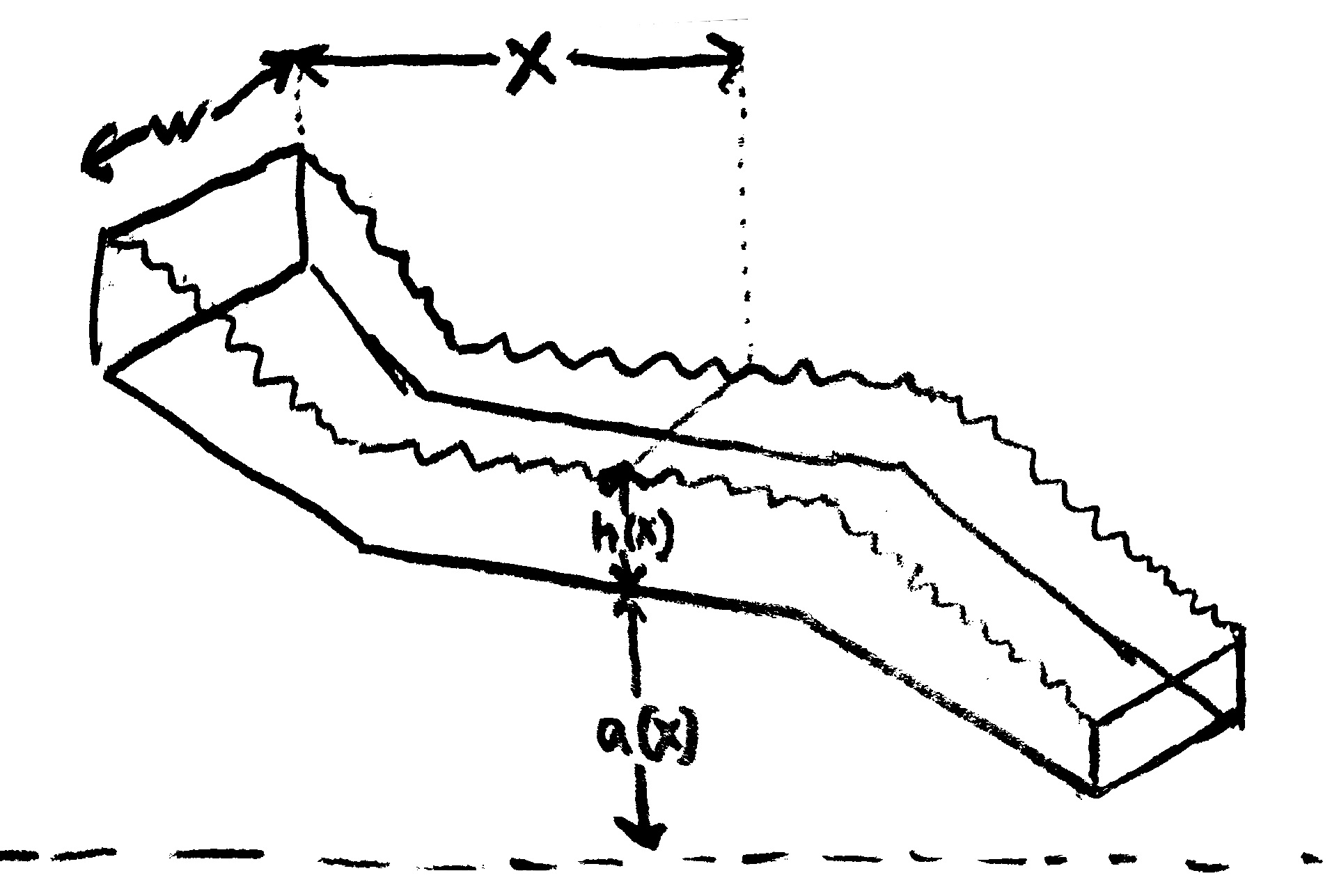Our constant discharge can be used to relate the water's depth to its velocity:. Where depth increases, velocity must decrease, and vice versa. Otherwise more water would pass through some parts of the river than others, which wouldn't make any sense. This simple fact already rules out option a. from the thought experiment.

To make more quantitative statements, we'll estimate the rate of change in the water's kinetic energy with respect todue to two net horizontal forces: the riverbed altitude changing and the water depth changing (). Note that all derivatives here are with respect to, not time.

Accounting for the riverbed altitude change is simple: as water vertically translates down at a rate of, gravity increases the water's kinetic energy at a rate ofGravity also has the effect of creating water pressure. Water at distanceabove the riverbed hasdistance of water above it, weighing down. For an area, the above water has weight, creating a pressure of. As the water depth changes, the pressure atabove riverbed changes as. Since water exerts force in all directions equally, this creates a horizontal pressure gradient, doing work at a rate ofIn total the water cell's kinetic energy increases at a rate ofKinetic energy is,, and, soAnd since our river has constant discharge,and. With a bit of work, we plug this in and solve forto get a dope ordinary differential equation (ODE):That denominator could be 0 - oh no! Don't worry, though. This is the right equation. It turns out the two cases - whetheror equivalently- have huge implications for the river's behavior.

• The slow case,, is called subcritical. The water is slow and deep enough that it can gain energy by speeding up and contracting its depth.
• The fast case,, is called supercritical. The water moves quickly enough that it can briefly force water ahead of it up a slope. Fun fact - supercritical water is moving fast enough that waves only propagate forwards relative to the ground. This requires speeds of 3 to 10 m/s in a typical river.

When a river does transition between these sub- and supercritical cases,undergoes a cusp at the critical point.

Looking at our formula forin the usual subcritical case, we can see that, so. In other words, if the riverbed is rising at a certain rate, the depth shrinks faster, so the top of the water drops. And since the depth shrinks, the velocity increases. Therefore the (typically) correct answer to the original thought experiment is b. In the supercritical case, we see that, so, which means that c. is also possible.

### Adding in Friction

The ODE we got without friction was lovely, but unfortunately friction always makes things uglier. We could account for friction against the land and/or friction within liquid. But we assumed from the beginning that the water has 0 viscosity, and viscosity is just friction within a liquid, so it makes sense to ignore that effect.

We'll take a simplistic approach: the frictional forceacting against the flow of water is proportional to

• ratio of contact perimeter with land to cross-sectional area, and
•.The motivation foris partly empirical, partly so that math is still nice, and partly intuitive. If a particle movestimes faster, it is likely to get intotimes more collisions attimes higher speed, resulting inas much energy loss.

In any case, after adding this term to our equation forand solving, we get a new ODE for:This ODE isn't linear, autonomous, or even homogeneous, so we don't have any hope of solving it. But we can use it numerically (as I did in the visualization above). And we can learn some interesting things about rivers with a constant slope!

### Special Case: Constant Slope River

In the case that the riverbed slopeat all, our ODE becomes autonomous:Without solving it, we can already glean one fact: if(any downhill river, as most are), there is a solution where the water depth stays a constantwith.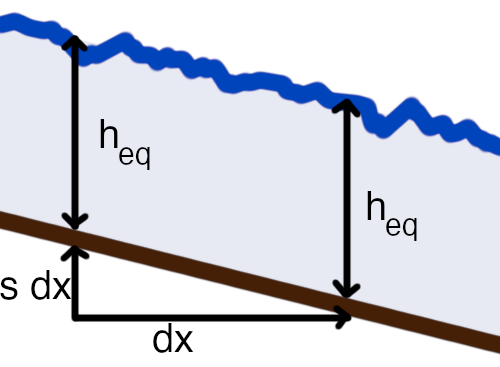Solving for, we get:Wow, we found a (depressed) cubic formula in real life! Instead of solving its hideous roots, let's just consider two cases: a narrow gorge (where) and a bayou (where):There are so many interesting points you can draw from these.

• In a narrow gorge, as water flow increases, the water doesn't move any faster - instead it just gets deeper.
• In a bayou, water speed increases with only the cube root of downhill slope, whereas in a narrow gorge, it increases more elastically with the square root.

Reducing the ODE to an implicit function ofis technically possible with partial fractions, but gives an equation far too complicated to be useful. Instead, let's consider an even easier case: a flat river.

### Extra Special Case: Flat River

How can a riverbed be flat? Wouldn't the river just stand still? Not necessarily - the water can actually propel itself forward a good distance by eating into its own potential energy. As the water moves forward, its depth decreases and it speeds up. This may sound strange, but consider that last 170km of the Mississippi drops by only 1cm per km - a 0.001% grade. Many rivers have sections like this, such as the Danube descending at less than 0.003% grade for 450km between Budapest and Belgrade.

With a flat river, we can solve the ODE to get an implicit function of:We can't solve forexplicitly, but it looks like this in the subcritical case:Asdrops to(the critical point), the water reaches it lowest possible energy state (balancing potential and kinetic energy), so it can no longer shorten itself to fight friction. In other words, a river can have a perfectly flat section and still move, but only for a moderate distance.

In the case that a supercritical river hits a flat section at high speed, it will also be able to progress for a short distance before it loses its speed and pushes backward. Imagine a fast hose aimed at a narrow channel before any of the water starts to roll backwards:The cusp we see here is a type of hydraulic jump. This video does a great job demonstrating them.

### Intermittent River Reversals

Because some real rivers are nearly flat, their direction can actually be reversed under the right circumstances. The Mississippi and Chicago rivers have both reversed because of storm surges. Storm surges increased water depth toward the mouth end of the river, pushing the water upstream via the higher water's potential energy (the subcritical case). During Hurricane Isaac, the reversed flow on the Mississippi was nearly twice as great as normal and lasted for 24 hours.

But I think the most interesting incident was the 1812 earthquake that caused the Mississippi to reverse for 3 days. From what I can gather, the earthquake didn't change the overall slope of the river - instead it just opened up new depressions and cavities for the river to flow into. The biggest of these was Reelfoot Lake, which was formerly dry land, but remains a lake to this day. Since the southern part of the river is essentially flat, the depth of the river exceeded its altitude change over hundreds of kilometers, so the water was able to flow backwards to fill these new depressions.

### Critical Behavior

Ok, so there are sub- and supercritical flows, and I've hinted that you can transition between them, but there's still something unsettling about the ODE. Once you hit the cusp where the denominator is 0, the ODE seems to give no possible way forward. In other words, once the water is out of potential and kinetic energy to fight friction, the equation seems unsatisfiable. But this doesn't match our intuition of some rivers, where a slow-moving lake can give way to a fast-moving cascade and vice versa. There are two reasons for this.

First, there is a cool way for the ODE to exhibit transcritical flow. The trick is that the numerator and denominator need to cross 0 at the exact same time; typically when the riverbed descends at the exact point when the water runs out of energy to burn. This is actually feasible because real rivers aren't exactly steady-state. An empty lakebed will fill up until it reaches the point where water can drain from it at an exactly critical rate. In the interactive visualization above, I did some tricks to make it easier to get this critical behavior.

Second, these equations ignore turbulence. This is of greatest importance in more general supercritical to subcritical transitions, where different layers of water move at different speeds (see below diagram). It's a limitation of this Saint Venant approach that some works aim to address.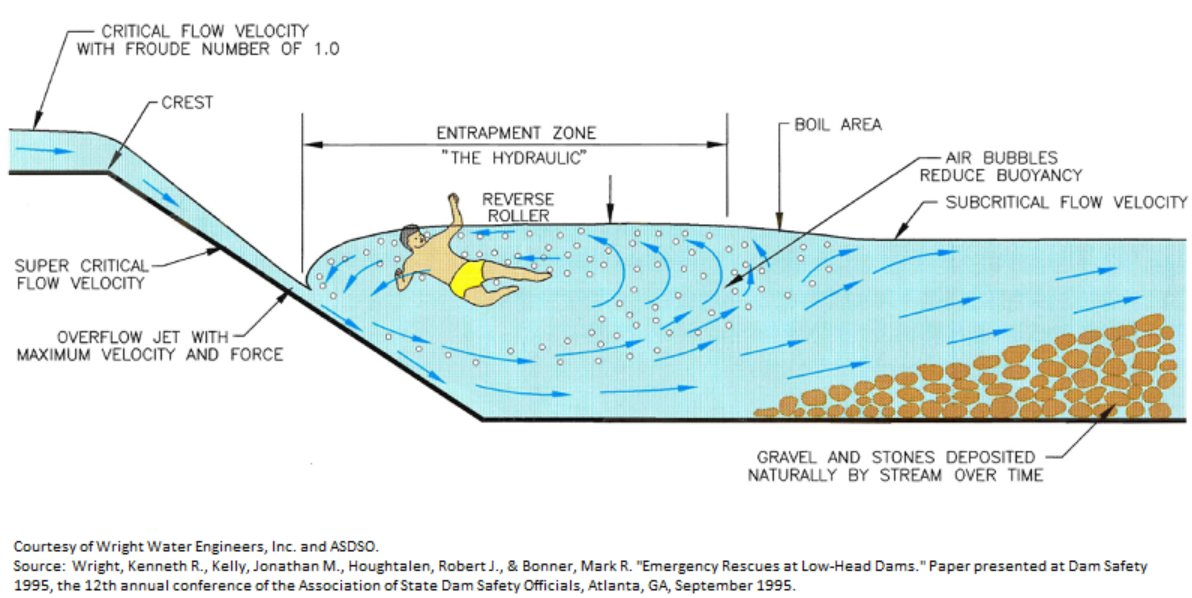### Final Morsels

With no mathematical justification: my collection of soothing river videos.

Thought Experiment Answer: typically b., but c. is also possible in very fast water.